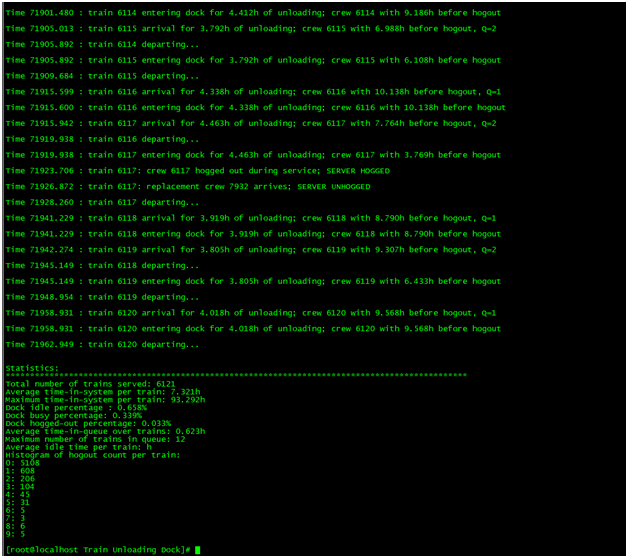# Train simulation assignment sample

Assignments on train simulation can be difficult to deal with especially because they require students to put in quite an amount of work. We help you with these assignments so you can have plenty of time to work on other school projects.

## Train simulation

Computer Simulation

Note: you CANNOT use CSIM – must use a non-simulation language

In this assignment, you will write a simulation of a train unloading dock. Trains arrive at the
station as a Poisson process on average once every 10 hours. Each train takes between 3.5 and 4.5
hours, uniformly at random, to unload. If the loading dock is busy, then trains wait in a first-come,
time passes between the departure of one train and the entry of the next train (if any) into the
There are a number of complications. Each train has a crew that, by union regulations, cannot work
more than 12 hours at a time. When a train arrives at the station, the crew’s remaining work time is
uniformly distributed at random between 6 and 11 hours. When a crew abandons their train at the
end of their shift, they are said to have “hogged out”. A train whose crew has hogged out cannot be
moved, and so if a hogged-out train is at the front of the queue and the train in front finishes
other trains cannot be used). Furthermore, a train that is already in the loading dock cannot be
unloaded in the absence of its crew, so once the crew hogs out, unloading must stop temporarily
until the next crew arrives for that train. This means that the unloading dock can be idle even if
there is a train in it, and even if it is empty and a (hogged-out) train is at the front of the queue.
Once a train’s crew has hogged out, the arrival of a replacement crew takes between 2.5 and 3.5
hours, uniformly at random. However, due to union regulations, the new crew’s 12-hour clock
starts ticking as soon as they are called in for replacement (i.e., at the instant the previous crew
hogged out); i.e., their 2.5-3.5 hour travel time counts as part of their 12-hour shift.
You will simulate for 72,000 hours, and output the following statistics at the end:
1. Total number of trains served.
2. Average and maximum of the time-in-system over trains.
3. The percentage of time the loading dock spent busy, idle, and hogged-out (does this add to
100%? Why or why not?)
4. Time average and maximum number of trains in the queue.
5. A histogram of the number of trains that hogged out 0, 1, 2, etc times.

Input Specification: create a makefile that builds your program, and your program
should take either one or two command-line arguments. When given only one argument, your
program should read that argument as the file path to the pre-determined train arrival schedule
(described below). When given two arguments, the first argument must be the average train interarrival time, and the second argument must be the total simulation time. Thus, for example, if your
program is written in C and compiled to an executable called “train”, then to run it with the default
parameters above, I should be able to run it on my Unix command line as:
% make
% ./train 10.0 72000
or
% make
% ./train schedule.txt
If you are using a language that does not run like the above (e.g. Python “python train.py 10.0
72000.0”) create a shell script or program wrapper that takes in the arguments and runs your code
as above. Also, if you are using a language that does not require building/compiling (e.g. Python),
just create a makefile with no targets:
target: ;
The pre-determined train arrival schedule contains three space-delimited columns: arrival times,
unloading times, and remaining crew hours (in that order), with each arrival event on a new line:
0.02013 3.70 8.92
8.12 4.12 10.10
12.52 3.98 7.82
1210.0 4.12 9.21
The simulation ends after the last train has departed.

Output Specification: Your program should print one line for every event that gets called. I want
to be able to follow what’s happening in your code. Each train and each crew should be assigned
an increasing integer ID. The final statistics should come after the simulation output and closely
match the specified format. Output lines should be identical the following example (except the
numbers, of course, which will be different for each run):
crew 0 with 7.80h before hogout (Q=0)
crew 0 with 7.80h before hogout
crew 1 with 6.12h before hogout (Q=1)
Time 3.72: train 0 departing (Q=1)
crew 1 with 2.96h before hogout
Time 6.68: train 1 crew 1 hogged out during service (SERVER HOGGED)
Time 9.43: train 1 replacement crew 2 arrives (SERVER UNHOGGED)
Time 1234.00: simulation ended
Statistics
———-
Total number of trains served: 512
Average time-in-system per train: 3.141593h
Maximum time-in-system per train: 6.283186h
Dock idle percentage: 64.00%
Dock busy percentage: 32.00%
Dock hogged-out percentage: 16.00%
Average time-in-queue over trains: 2.718282h
Maximum number of trains in queue: 2
Average idle time per train: 0.707107h
Histogram of hogout count per train:
: 512
: 128
: 32
… (show as many bins as necessary)
Numbers can have any number of decimal places, but noscientific notation. A script to automatically check the output will be provided feedback on I/Ospecification compliance.

Solution

commons.h

#ifndef COMMONS_H_

#define COMMONS_H_

#include

#include

#include

#include “entities.h”

/////////////////////////

// useful declarations //

/////////////////////////

// event priority queue comparator

structbyTime

{

bool operator()(const Event& lhs, const Event&rhs) const

{

returnlhs.get_e_time() >rhs.get_e_time();

}

};

// random generators

externstd::mt19937 rng;

externstd::uniform_real_distributioninter_arr_time;

externstd::uniform_real_distributionc_time;

externstd::uniform_real_distributionarr_time;

extern double average_oc;

extern double hours_simulated;

externinttotal_crew_in_work;

externintnum_trains_arrived;

extern double total_time_simulated;

externstd::queuearrival_queue;

externstd::priority_queue, byTime>event_queue;

#endif /* COMMONS_H_ */

entities.cpp

#include

#include

#include “entities.h”

#include “commons.h”

using namespace std;

//////////

// Crew //

//////////

void Crew::setup(int id, double work_time)

{

cid = id;

remaining_work_time = work_time;

}

int Crew::get_cid()

{

returncid;

}

double Crew::get_wt()

{

returnremaining_work_time;

}

void Crew::update_wt(double time_worked)

{

if (time_worked

{

remaining_work_time -= time_worked;

}

else

{

remaining_work_time = 0;

}

}

///////////

// Train //

///////////

void Train::setup(int id, intpos, double unload_time, double t_arrival, Crew c)

{

tid = id;

current_pos_in_queue = pos;

time_needed_to_unload = unload_time; // will take between 3.5 and 4.5 hours uniformly at random

time_arrived = t_arrival;

unload_start_time = time_arrived + 0.0001; // default prediction with slight delay

assigned_c = c;

total_time_worked = assigned_c.get_wt(); // default prediciton of how long crews will work

time_departed = unload_start_time + time_taken_to_unload; // default prediction of when the train will depart (IF THERE IS NO HOGOUT and UNLOADS as soon as the train arrives)

// initialize hog times with zero

hogged_stime = 0;

unhogged_stime = 0;

}

void Train::change_pos_in_queue(int p)

{

current_pos_in_queue -= p;

}

void Train::calc_dep_time()

{

// check if train can get unloaded with the current crew’s remaining time

doublecrew_work_time = assigned_c.get_wt();

if (crew_work_time

{

// TRAIN WILL GET HOGGED OUT (THIS IS FOR WHEN TRAIN MOVES INTO DOCK — NOT WHILE IN QUEUE)

hogged_stime = this->get_crew_remaining_time();

this->queue_HOGGED_event();

// update various times

// find replacement crew

doublerep_time_taken = arr_time(rng);

// replacement crew found… update

total_time_worked += rep_time_taken;

Crew rep_crew;

doublenew_time = c_time(rng) – rep_time_taken;

total_crew_in_work++;

rep_crew.setup(total_crew_in_work, new_time);

// assign crew to train and update crew_wt

assigned_c = rep_crew;

crew_work_time = assigned_c.get_wt();

total_time_worked += crew_work_time;

// create UNHOGGED event and queue it

unhogged_stime = hogged_stime + rep_time_taken;

this->queue_UNHOGGED_event();

// increment number of times hogged out

times_hogged_out++;

}

// update time_departed

}

double Train::get_dep_time()

{

returntime_departed;

}

double Train::get_arr_time()

{

returntime_arrived;

}

{

}

{

}

double Train::get_crew_remaining_time()

{

returntotal_time_worked + time_arrived;

}

double Train::get_unhogged_stime()

{

returnunhogged_stime;

}

int Train::get_times_hogged_out()

{

returntimes_hogged_out;

}

void Train::reset_crew_remaining_time()

{

assigned_c.update_wt(assigned_c.get_wt());

}

{

{

double td = (t – unload_start_time) + 0.0001; // add slight delay

if (times_hogged_out == 0)

{

assigned_c.update_wt(td);

}

else

{

{

}

else

{

}

}

}

}

string Train::arrival_prompt()

{

ostringstream res;

res<< fixed <

res<< “Time ” <

res<< ” arrival for ” <

res<< ” with ” <

returnres.str();

}

string Train::service_prompt()

{

ostringstream res;

res<< fixed <

res<< “Time ” <

res<< ” entering dock for ” <

res<< ” with ” <

res<

returnres.str();

}

string Train::dep_prompt()

{

ostringstream res;

res<< fixed <

res<< “Time ” <

res<< ” departing…” <

returnres.str();

}

string Train::hog_prompt()

{

ostringstream h1;

h1 << fixed <

h1 << “Time “<

h1 << “: crew ” <

h1 <

return h1.str();

}

string Train::unhog_prompt()

{

ostringstream h2;

h2 << fixed <

h2 << “Time “<

h2 << “: replacement crew ” <

h2 <

return h2.str();

}

void Train::queue_arrival_event()

{

Event e(time_arrived, tid, ARRIVAL, this->arrival_prompt());

event_queue.push(e);

}

{

event_queue.push(e);

}

void Train::queue_dep_event()

{

//this->calc_dep_time();

Event e(time_departed, tid, DEPARTURE, this->dep_prompt());

event_queue.push(e);

}

void Train::queue_HOGGED_event()

{

Event e(hogged_stime, tid, HOGGED, this->hog_prompt());

event_queue.push(e);

}

void Train::queue_UNHOGGED_event()

{

Event e2(unhogged_stime, tid, UNHOGGED, this->unhog_prompt());

event_queue.push(e2);

}

void Train::queue_REPLACE_event()

{

doublecrew_work_time = assigned_c.get_wt();

// create HOGGED OUT event and queue it

hogged_stime = this->get_crew_remaining_time();

this->queue_HOGGED_event();

// find replacement crew

doublerep_time_taken = arr_time(rng);

// replacement crew found… update

total_time_worked += rep_time_taken;

Crew rep_crew;

doublenew_time = c_time(rng) – rep_time_taken;

total_crew_in_work++;

rep_crew.setup(total_crew_in_work, new_time);

// assign crew to train and update crew_work_time

assigned_c = rep_crew;

crew_work_time = assigned_c.get_wt();

total_time_worked += crew_work_time;

// create UNHOGGED event and queue it

unhogged_stime = hogged_stime + rep_time_taken;

this->queue_UNHOGGED_event();

// increment number of times hogged out

times_hogged_out++;

}

///////////

// Event //

///////////

Event::Event(double t, int id, event_statees, string m)

{

e_time = t;

tid = id;

e_state = es;

e_msg = m;

}

void Event::decrease_tid()

{

tid–;

}

string Event::get_event() const

{

returne_msg;

}

double Event::get_e_time() const

{

returne_time;

}

event_state Event::get_e_state() const

{

returne_state;

}

entities.h

#ifndef ENTITIES_H_

#define ENTITIES_H_

#pragma once

#include

#include

enumevent_state

{

};

/////////////////////

// system entities //

/////////////////////

class Crew

{

private:

intcid;

doubleremaining_work_time = 12;

public:

void setup(int id, double work_time);

intget_cid();

doubleget_wt();

voidupdate_wt(double time_worked); // updates remaining_work_time with time_worked (resets to 0 if its greater — meaning crew no longer works)

};

class Train

{

private:

inttid;

intcurrent_pos_in_queue;

doubletime_arrived;

doubletime_departed;

double unload_start_time; // different from time_arrived in that it could’ve been in queue but didn’t start unloading until after the train in front is done

doublehogged_stime;

doubleunhogged_stime;

doubletotal_time_worked;

inttimes_hogged_out = 0; // has assigned default value of 0

Crew assigned_c;

public:

void setup(int id, intpos, double unload_time, double t_arrival, Crew c);

voidcalc_dep_time(); // calculates departure time (prediction)

voidchange_pos_in_queue(int p);

doubleget_dep_time();

doubleget_arr_time();

doubleget_crew_remaining_time();

doubleget_unhogged_stime();

intget_times_hogged_out();

voidreset_crew_remaining_time();

std::string arrival_prompt();

std::string service_prompt();

std::string dep_prompt();

std::string hog_prompt();

std::string unhog_prompt();

voidqueue_arrival_event();

voidqueue_dep_event();

voidqueue_HOGGED_event();

voidqueue_UNHOGGED_event();

voidqueue_REPLACE_event();

};

class Event

{

private:

doublee_time;

inttid;

event_statee_state;

std::string e_msg;

public:

Event(double t, int id, event_statees, std::string m);

voiddecrease_tid();

std::string get_event() const;

doubleget_e_time() const;

event_stateget_e_state() const;

};

#endif /* ENTITIES_H_ */

main.cpp

#include

#include

#include

#include

#include

#include

#include

#include “entities.h”

#include “commons.h”

using namespace std;

////////////////////////

// Extern definitions //

////////////////////////

// random generators

mt19937rng(random_device { }());

uniform_real_distributioninter_arr_time(0.0, 1.0);

uniform_real_distributionarr_time(2.5, 3.5);

uniform_real_distributionc_time(6.0, 11.0);

// Extern variables

doubleaverage_oc = 0.0;

doublehours_simulated = 0.0;

inttotal_crew_in_work = 0;

intnum_trains_arrived = 0;

doubletotal_time_simulated = 0.0;

// the important queues

queuearrival_queue;

queuearrival_queue_copy;

priority_queue, byTime>event_queue;

maphogged_histogram;

////////////////////

// main functions //

////////////////////

template

voidsimulate_event_queue(T& q)

{

double CURR_TIME = 0.0;

double TIME_IDLE = 0.0;

double TIME_BUSY = 0.0;

double TIME_HOGGED = 0.0;

bool BUSY = false;

vectortime_spent_in_queue;

vectortime_intervals;

vectortrains_in_queue;

intnum_trains_in_queue = 0;

while (!q.empty())

{

string m = q.top().get_event();

event_state e = q.top().get_e_state();

double _t = q.top().get_e_time() – CURR_TIME;

// check event states and gather stats

switch (e)

{

case (ARRIVAL):

// maintain how many trains are in queue

num_trains_in_queue++;

// update queue history and time intervals

trains_in_queue.push_back(num_trains_in_queue);

time_intervals.push_back(q.top().get_e_time());

// update its output string

m += (“, Q=” + to_string(num_trains_in_queue) + “\n”);

if (!BUSY)

{

TIME_IDLE += _t;

BUSY = true;

}

else

{

TIME_BUSY += _t;

}

break;

case (DEPARTURE):

num_trains_in_queue–;

BUSY = false;

TIME_BUSY += _t;

// add time spent in queue for each train

time_spent_in_queue.push_back(q.top().get_e_time() – arrival_queue_copy.front().get_arr_time());

arrival_queue_copy.pop();

// update queue history and time intervals

trains_in_queue.push_back(num_trains_in_queue);

time_intervals.push_back(q.top().get_e_time());

break;

case (HOGGED):

BUSY = false;

TIME_BUSY += _t;

break;

case (UNHOGGED):

BUSY = true;

TIME_HOGGED += _t;

TIME_IDLE += _t;

break;

// do nothing

break;

}

// print out event

cout<< m <

// update current time

CURR_TIME = q.top().get_e_time();

q.pop();

}

// some final calculations

double sum = accumulate(time_spent_in_queue.begin(), time_spent_in_queue.end(), 0.0);

doubleavg_t = sum / num_trains_arrived;

doubleq_sum = 0;

for (size_t i = 0; i

{

q_sum += (time_intervals[i + 1] – time_intervals[i]) * trains_in_queue[i];

}

doubleq_avg = q_sum / CURR_TIME;

// OUTPUT RESULTS

cout<< fixed <

cout<

cout<< “Statistics: ” <

cout<< string(95, ‘*’) <

// Q1

cout<< “Total number of trains served: ” <

// Q2

cout<< “Average time-in-system per train: ” <

cout<< “Maximum time-in-system per train: ” << *max_element(time_spent_in_queue.begin(), time_spent_in_queue.end()) << “h” <

// Q3

cout<< “Dock idle percentage : ” << TIME_IDLE / CURR_TIME << “%” <

cout<< “Dock busy percentage: ” << TIME_BUSY / CURR_TIME << “%” <

cout<< “Dock hogged-out percentage: ” << TIME_HOGGED / CURR_TIME << “%” <

// Q4

cout<< “Average time-in-queue over trains: ” <

cout<< “Maximum number of trains in queue: ” << *max_element(trains_in_queue.begin(), trains_in_queue.end()) <

// Q5

cout<< “Histogram of hogout count per train: ” <

for (auto&kv : hogged_histogram)

{

cout<

}

cout<

}

void initialize(double aoc, double hs)

{

average_oc = 1 / aoc;

hours_simulated = hs;

// start simulation

while (1)

{

// First, calculate inter arrival time between trains (with lambda = average_oc)

double u = inter_arr_time(rng);

doubletrain_arrival_time = -(log(u)) / average_oc;

total_time_simulated += train_arrival_time;

// check exit condition

if (total_time_simulated>hours_simulated)

{

break;

}

// setup train and its crew

Train curr_train;

inttid = num_trains_arrived;

intcid = total_crew_in_work;

intpos = arrival_queue.size();

doublecrew_wt = c_time(rng);

doublet_arrival = total_time_simulated;

Crew c;

c.setup(cid, crew_wt);

// train is ready — add to arrival queue and event queue

arrival_queue.push(curr_train);

num_trains_arrived++;

total_crew_in_work++;

}

// maintain a copy of the arrival queue (used later for statistics purposes)

arrival_queue_copy = arrival_queue;

}

voidupdate_event_queue()

{

deque dock;

doublenext_event_time = 0.0;

intts = 0;

while (ts != num_trains_arrived)

{

Train curr_train;

if (dock.empty() && !arrival_queue.empty())

{

dock.push_back(arrival_queue.front());

dock.front().queue_arrival_event();

arrival_queue.pop();

dock.front().calc_dep_time();

}

else

{

curr_train = dock.front();

// will a new train come while the train in front is unloading?

(!arrival_queue.empty()) ?

next_event_time = min(curr_train.get_dep_time(), arrival_queue.front().get_arr_time()) : next_event_time = curr_train.get_dep_time();

// will any trains in queue (if size > 1) HOG OUT before the a new train arrives or train in front departs?

if (dock.size() > 1)

{

for (size_t i = 1; i

{

if (dock[i].get_crew_remaining_time()

{

dock[i].queue_REPLACE_event();

}

}

}

if (next_event_time == curr_train.get_dep_time())

{

dock.front().queue_dep_event();

inth_key = dock.front().get_times_hogged_out();

int count = hogged_histogram.count(h_key);

if (count > 0)

{

// key found

hogged_histogram.at(h_key) += 1;

}

else

{

// no key (create a new pair)

hogged_histogram.insert(pair(h_key, 1));

}

dock.pop_front();

if (!dock.empty())

{

dock.front().calc_dep_time();

}

ts++;

}

else if (next_event_time == arrival_queue.front().get_arr_time())

{

dock.push_back(arrival_queue.front());

dock.back().queue_arrival_event();

arrival_queue.pop();

}

}

}

}

int main(intargc, char *argv[])

{

initialize(atoi(argv), atoi(argv));

update_event_queue();

simulate_event_queue(event_queue);

return 0;

Makefile

#Set all your object files (the object files of all the .c files in your project, e.g. main.omy_sub_functions.o )

OBJ = main.oentities.o

#Set any dependant header files so that if they are edited they cause a complete re-compile (e.g. main.hsome_subfunctions.hsome_definitions_file.h ), or leave blank

DEPS = commons.hentities.h

#Any special libraries you are using in your project (e.g. -lbcm2835 -lrt `pkg-config –libs gtk+3.0` ), or leave blank

LIBS =

#Set any compiler flags you want to use (e.g. -I/usr/include/somefolder `pkg-config –cflags gtk+3.0` ), or leave blank

CFLAGS = -std=cﭝ -Wall

#Set the compiler you are using ( gcc for C or g for C )

CC = g

#Set the filename extensiton of your C files (e.g. .c or .cpp )

EXTENSION = .cpp

#define a rule that applies to all files ending in the .o suffix, which says that the .o file depends upon the .c version of the file and all the .h files included in the DEPS macro. Compile each object file

%.o: %\$(EXTENSION) \$(DEPS)

\$(CC) -c -o \$@ \$< \$(CFLAGS)

#Combine them into the output file

#Set your desired exe output file name here

train: \$(OBJ)

\$(CC) -o \$@ \$^ \$(CFLAGS) \$(LIBS)

#Cleanup

.PHONY: clean

clean:

rm -f *.o *~ core *~

1 How to Run The Program
Build: using makefile. Type make
Run: ./train
Eg: ./train 12.0 72000. This mean will be train for one entries in 12 hours, and will be train in 72000 hours è Total entries will be train: 72000 / 12 ≈ 6000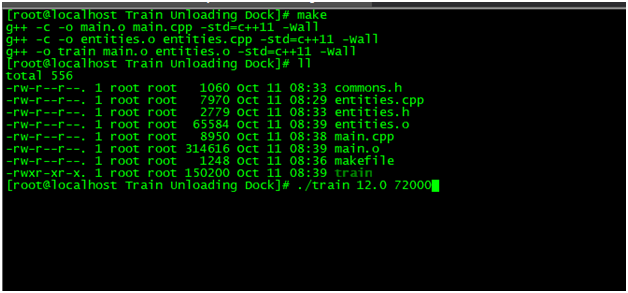Figure 1‑1: Compile and Run the Program

#### 2 Entitries

2.1 Crew Class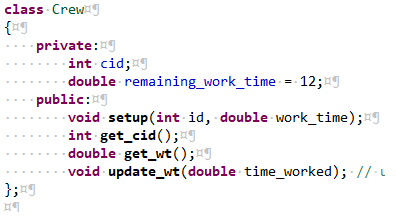Figure 2‑1: Crew Class

2.2 Train Class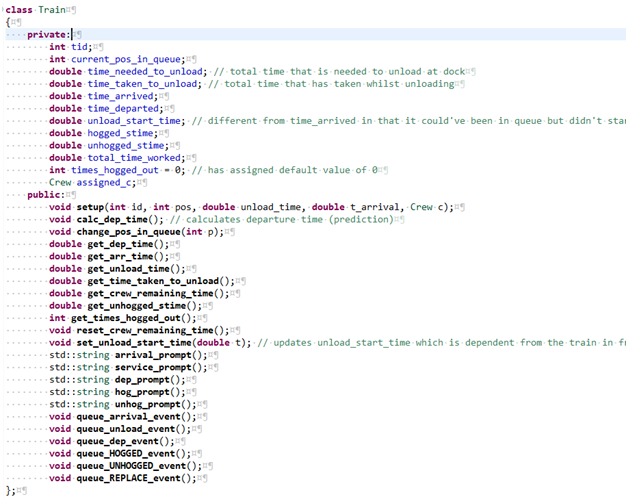Figure 2‑2: Train Class

2.3 Event
Figure 2‑3: Event Class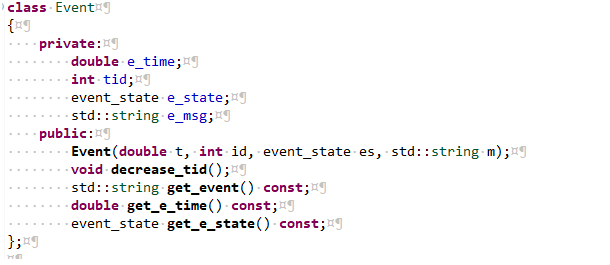#### 3 Initialize data

Function: voidinitialize(doubleaverage_oc, doublehours_simulated)
First, calculate inter arrival time between trains(with lambda = average_oc, average_oc = 1 /average train inter-arrival time). When total simulator time come to limit. The program exit.
Create Crew object with ID crew working time is random in 6.0(hours) to 11(hours).
Create Train object with unload time is random in 3.5(hours) to 4.5(hours)
Push the Train object to the ARRIVAL QUEUE

#### 4 Update Event Queue

Function: voidupdate_event_queue()
Base on time of each Train object. We calculate depart time of each Object. Then we create the event with state for ARRIVAL, UNLOAD, DEPARTURE, HOGGED or UNHOGGED. And push to Event queue
Event Queue is PRIORITY QUEUE sort by event process time. priority_queue, byTime>event_queue;